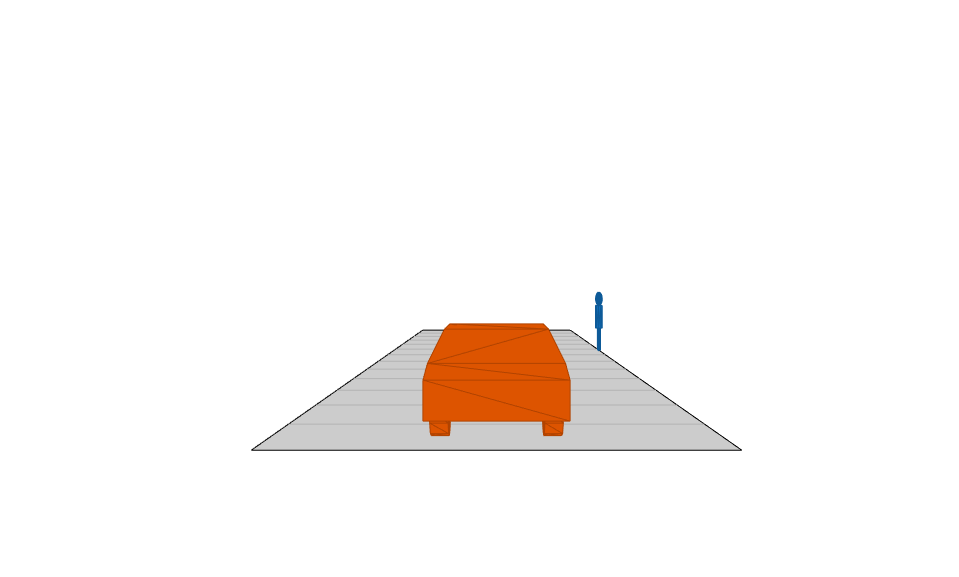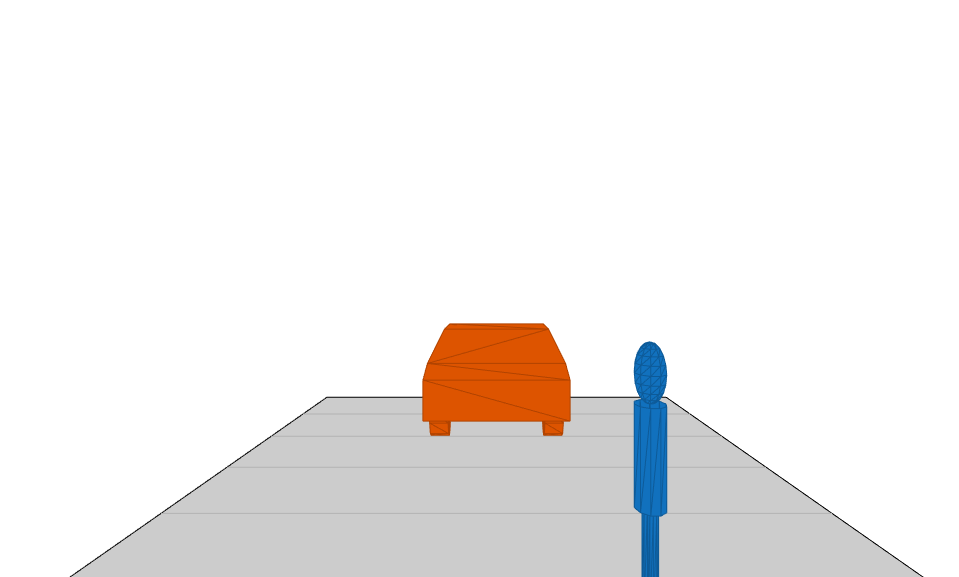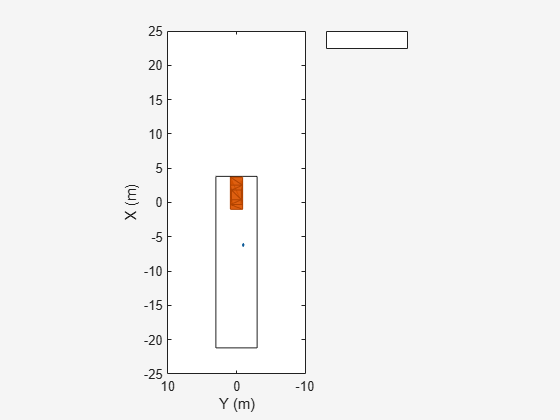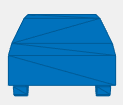# plotMesh

Display object meshes on bird's-eye plot

## Syntax

``plotMesh(mPlotter,vertices,faces)``
``plotMesh(mPlotter,vertices,faces,'Color',colors)``

## Description

````plotMesh(mPlotter,vertices,faces)` displays meshes composed of the specified vertices and faces on a bird's-eye plot. To obtain the mesh vertices and faces of an object in a driving scenario, use the `targetMeshes` function. The mesh plotter, `mPlotter`, is associated with a `birdsEyePlot` object and configures the display of the meshes.The bird's-eye plot assigns a different color to each actor, based on the default color order of `Axes` objects. For more details, see the `ColorOrder` property for `Axes` objects.To remove all meshes associated with mesh plotter `mPlotter`, call the `clearData` function and specify `mPlotter` as the input argument.```

example

````plotMesh(mPlotter,vertices,faces,'Color',colors)` specifies the colors of the meshes.```

## Examples

collapse all

Display actors in a driving scenario by using their mesh representations instead of their cuboid representations.

Create a driving scenario, and add a 25-meter straight road to the scenario.

```scenario = drivingScenario; roadcenters = [0 0 0; 25 0 0]; road(scenario,roadcenters); ```

Add a pedestrian and a vehicle to the scenario. Specify the mesh dimensions of the actors using prebuilt meshes.

• Specify the pedestrian mesh as a `driving.scenario.pedestrianMesh` object.

• Specify the vehicle mesh as a `driving.scenario.carMesh` object.

```p = actor(scenario,'ClassID',4, ... 'Length',0.2,'Width',0.4, ... 'Height',1.7,'Mesh',driving.scenario.pedestrianMesh); v = vehicle(scenario,'ClassID',1, ... 'Mesh',driving.scenario.carMesh); ```

Add trajectories for the pedestrian and vehicle.

• Specify for the pedestrian to cross the road at 1 meter per second.

• Specify for the vehicle to follow the road at 10 meters per second.

```waypointsP = [15 -3 0; 15 3 0]; speedP = 1; smoothTrajectory(p,waypointsP,speedP); wayPointsV = [v.RearOverhang 0 0; (25 - v.Length + v.RearOverhang) 0 0]; speedV = 10; smoothTrajectory(v,wayPointsV,speedV) ```

Add an egocentric plot for the vehicle. Turn the display of meshes on.

```chasePlot(v,'Meshes','on') ```Create a bird's-eye plot in which to display the meshes. Also create a mesh plotter and lane boundary plotter. Then run the simulation loop.

1. Obtain the road boundaries of the road the vehicle is on.

2. Obtain the mesh vertices, faces, and colors of the actor meshes, with positions relative to the vehicle.

3. Plot the road boundaries and actor meshes on the bird's-eye plot.

4. Pause the scenario to allow time for the plots to update. The chase plot updates every time you advance the scenario.

```bep = birdsEyePlot('XLim',[-25 25],'YLim',[-10 10]); mPlotter = meshPlotter(bep); lbPlotter = laneBoundaryPlotter(bep); legend('off') while advance(scenario) rb = roadBoundaries(v); [vertices,faces,colors] = targetMeshes(v); plotLaneBoundary(lbPlotter,rb) plotMesh(mPlotter,vertices,faces,'Color',colors) pause(0.01) end ```## Input Arguments

collapse all

Mesh plotter, specified as a `MeshPlotter` object. This object is stored in the `Plotters` property of a `birdsEyePlot` object and configures the display of meshes in the bird's-eye plot. To create this object, use the `meshPlotter` function.

Mesh vertices of each actor, specified as an N-element cell array, where N is the number of actors.

Each element in `vertices` must be a V-by-3 real-valued matrix containing the vertices of an actor, where:

• V is the number of vertices.

• Each row defines the 3-D (x,y,z) position of a vertex. When you use the `targetMeshes` function to obtain mesh vertices, the vertex positions are relative to the position of the actor that is input to that function. Units are in meters.

Mesh faces of each actor, specified as an N-element cell array, where N is the number of actors.

Each element in `faces` must be an F-by-3 integer-valued matrix containing the faces of an actor, where:

• F is the number of faces.

• Each row defines a triangle of vertex IDs that make up the face. The vertex IDs correspond to row numbers within `vertices`.

Suppose the first face of the `i`th element of `faces` has these vertex IDs.

`faces{i}(1,:)`
```ans = 1 2 3```

In the `i`th element of `vertices`, rows 1, 2, and 3 contain the (x, y, z) positions of the vertices that make up this face.

`vertices{i}(1:3,:)`
```ans = 3.7000 0.9000 0.8574 3.7000 -0.9000 0.8574 3.7000 -0.9000 0.3149```

Color of the mesh faces for each actor, specified as an N-by-3 matrix of RGB triplets. N is the number of actors and is equal to the number of elements in `vertices` and `faces`.

The `i`th row of `colors` is the RGB color value of the faces in the `i`th element of `faces`. The function applies the same color to all mesh faces of an actor.

An RGB triplet is a three-element row vector whose elements specify the intensities of the red, green, and blue components of the color. The intensities must be in the range [0, 1]. For example, `[0.4 0.6 0.7]`.

collapse all

### Meshes

In driving scenarios, a mesh is a triangle-based 3-D representation of an object. Mesh representations of objects are more detailed than the default cuboid (box-shaped) representations of objects. Meshes are useful for generating synthetic point cloud data from a driving scenario.

This table shows the difference between a cuboid representation and a mesh representation of a vehicle in a driving scenario.

CuboidMesh## Version History

Introduced in R2020b